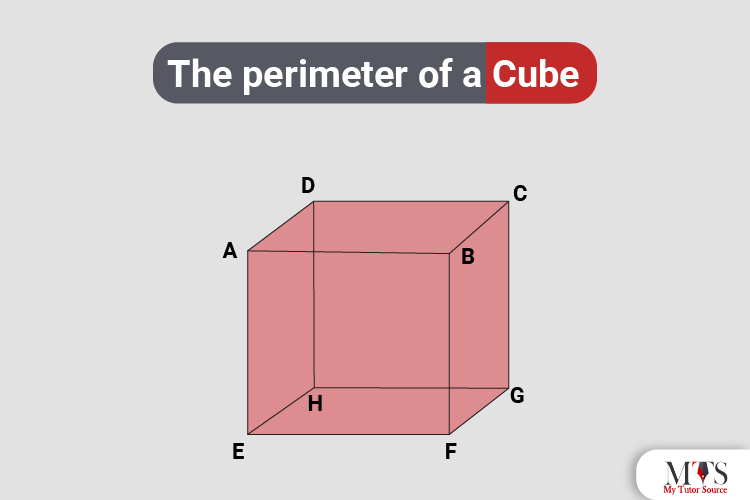# The perimeter of a Cube: Formulas, Examples, and More!Finding the perimeter of a cube is often mistaken as an ‘impossible’ task in Mathematics. Why? Well, because the cube is a three-dimensional figure, and the perimeter is only linked with two-dimensional objects. However, that is not the case. Calculating the perimeter of a cube might be one of the most difficult tasks of Mathematics, but not impossible.

A cube may not be a two-dimensional object itself, but it is a collection and sum of different two-dimensional shapes. Want to know how? Because all the 6 faces of the cube are square. If you are a tutor or a student confused about this concept, don’t worry. As always, we are here to the rescue!

In this post, we are going to give a detailed explanation and answer of how to find the perimeter of a cube with easy-to-understand formulas and examples. But before that, let us take a brief look at the definition of a cube to better understand the concept. Keep Reading!

## What is a Cube?

As we have briefly mentioned above, a cube is a three-dimensional shape and has an XYZ plane. It contains 8 verticals, 6 faces, and 12 edges. Also, all the faces and sides of the cube have a square shape and equal dimensions. Moreover, all the opposite edges of the cube will always be parallel to each other.

## How to Find the Perimeter of a Cube

Do you remember how to find a square’s perimeter? Yes, by summing up the lengths of all sides of the square. Similarly, you need to sum up all the separate sides of the cube to find its perimeter. These separate sides are also called the edges of the cube. Now, let us move forward with the formulas and examples!

### Formulas to Find the Perimeter of a Cube

Though nowadays we can find the perimeter through an online cube calculator tool, still, learning and understanding the formulas is essential for students.

You can find the perimeter of a cube by summing up all the edges and sides of the cube. Now, there are a total of 12 edges in a cube. Also, we know that all the 12 edges are going to have the same and equal length, right?

With that being said, the suitable and verified formula for the perimeter of a cube is:

p = 12L

L = length

p = perimeter

12 = edges of the cube

Each example mentioned below is solved and contains easy, step-by-step solutions.

Example # 1

A cube has 12 edges of 10 m each. What is the perimeter of the cube?

Solution:

Keeping in mind that L = 10, we apply the formula:
p = 12L
p = 12 (10) {Multiply}
p = 120

Hence, the perimeter of this cube is 120 m.

Example # 2

A cube has 12 edges of 28 m each. What is the perimeter of the cube?

Solution:

Keeping in mind that L = 28, we apply the formula:
p = 12L
p = 12 (28) {Multiply}
p = 336

Hence, the perimeter of this cube is 330 m.

Example # 3

A cube has 12 edges of 13 m each. What is the perimeter of the cube?

Solution:

Keeping in mind that L = 13, we apply the formula:
p = 12L
p = 12 (13) {Multiply}
p = 156

Hence, the perimeter of this cube is 156 m.

Example # 4

If the perimeter of a cube is 140 m, how long are its edges?

Solution:

Keeping in mind that p = 140, we apply the formula and obtain:
p = 12L
140 = 12L
L = 11.66

We divided the Length (140) by 12. Hence, the edges are 11.66 m long.

Example # 5

If the perimeter of a cube is 300 m, how long are its edges?

Solution:

Keeping in mind that p = 140 m, we apply the formula and obtain:
p = 12L
300 = 12L
L = 25

We divided the Length (300) by 12. Hence, the edges are 25 m long.

## Final Words

Now that you have gone through the entire post, we are sure you got your answer! See… Calculating the perimeter is possible, and we hope we kind of made it easy for you as well! If you have any more math-related queries, just scroll down our blog and we are sure you will find your answers!

Also, we would suggest you solve and practice as many questions as you can be related to the perimeter of a cube. This way, you will have a better understanding and absorption of the concept.

### FAQs for Perimeter of a Cube

#### Does all the sides of a cube have equal lengths?

Yes, all the sides of a cube will always have the equal and same length.

#### How do you calculate the perimeter of a square?

Just like other two-dimensional objects, you need to sum up the lengths of all sides of the square too to find the perimeter.

### Find Top Tutors in Your AreaWith over 3 years of experience in teaching, Chloe is very deeply connected with the topics that talk about the educational and general aspects of a student's life. Her writing has been very helpful for students to gain a better understanding of their academics and personal well-being. I’m also open to any suggestions that you might have! Please reach out to me at chloedaniel402 [at] gmail.com